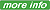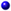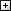Processing ......FreeComputerBooks.com Free Computer, Mathematics, Technical Books and Lecture Notes, etc.

Differential Equations and Dynamical Systems
Want to know the elevation (AMSL) at any spot all over world? Try GIS Visualizer.
:Numerical Computation BooksCalculus and Mathematical Analysis BooksFourier and Wavelet Transforms BooksGeometry and Topology BooksStochastic Differential Equations: Models and Numerics (Jesper Carlsson, et al)Elementary Differential Equations with Boundary Value Problems (William F. Trench)Elementary Differential Equations (William F. Trench)A Friendly Introduction to Differential Equations (Mohammed K A Kaabar)Notes on Diffy Qs: Differential Equations for Engineers ©2012 (Jiri Lebl)Complex and Adaptive Dynamical Systems: A Primer ©2010 (Claudius Gros)Linear Partial Differential Equations and Fourier Theory ©2010 (Marcus Pivato)Asymptotically Almost Periodic Solutions of Differential Equations ©2009 (David N. Cheban)Solvability of Nonlinear Singular Problems for Ordinary Differential Equations ©2009 (I. Rachunkova, et al)Qualitative Analysis of Nonlinear Elliptic Partial Differential Equations ©2008 (Vicentiu D. Radulescu)Introduction to The Theory of Functional Differential Equations ©2007 (N. V. Azbelev, V. P. Maksimov, et al)Qualitative Analysis of Delay Partial Difference Equations ©2007 (Binggen Zhang, Yong Zhou)Lectures on Analytic Differential Equations ©2007 (Yulij Ilyashenko, Sergei Yakovenko) 625 pagesOrdinary Differential Equations And Dynamical Systems ©2007 (Gerald Teschl)Impulsive Differential Equations and Inclusions ©2006 (M. Benchohra, et al) 370 pagesProceedings of the Conference on Differential & Difference Equations and Applications ©2006 (Ravi P. Agarwal)Discrete Oscillation Theory ©2005 (Ravi P. Agarwal, et al) 961 pagesExterior Differential Systems and Euler-Lagrange Partial Differential Equations ©2003 (Robert L. Bryant, et al)Existence, Multiplicity, Perturbation, and Concentration Results for Elliptic Problems ©2006 (Marco Squassina) 213 pagesStrange Attractors: Creating Patterns in Chaos ©1993-2000 (Julien C. Sprott)Difference Equations to Differential Equations: An Introduction to Calculus ©2000 (Dan Sloughter)An Introduction to Stochastic Differential Equations (Lawrence Craig Evans)Finite Difference and Spectral Methods for Ordinary and Partial Differential Equations ©1994 (Lloyd N. Trefethen)Notes on Differential Equations (Robert E. Terrell)Ordinary Differential Equations (Sheldon Newhouse)
Book CategoriesAll CategoriesRecent BooksIT Research LibraryMiscellaneous BooksComputer LanguagesComputer ScienceData Science/DatabasesElectronic EngineeringJava and Java EE (J2EE)Linux and UnixMathematicsMicrosoft and .NETMobile ComputingNetworking and CommunicationsSoftware EngineeringSpecial TopicsWeb Programming
Other Categories